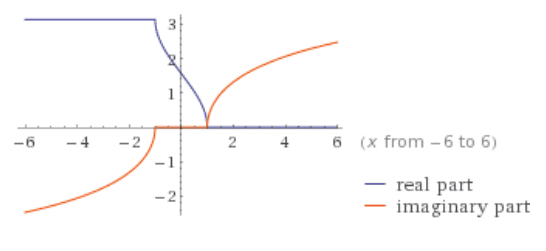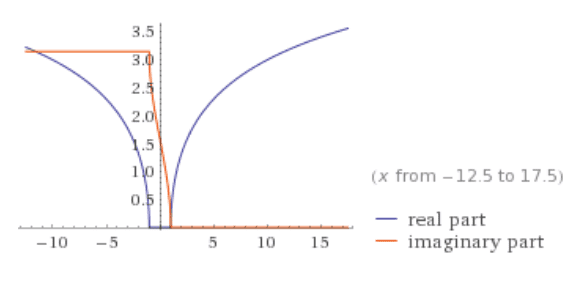# Graphs of inverse trigonometric vs inverse hyperbolic functions

• I
I noticed the graphs of ##y=\cos^{-1}x## and ##y=\cosh^{-1}x## are similar in the sense that the real part of one is the imaginary part of the other. This is true except when ##x<-1## where the imaginary part of ##y=\cos^{-1}x## is negative but the real part of ##y=\cosh^{-1}x## is positive.

I believe whether they are positive or negative is by a choice of convention. So why don't we define them to be both positive or both negative?

##\cos^{-1}x## graph: http://www.wolframalpha.com/input/?i=y=acos(x)##\cosh^{-1}x## graph: http://www.wolframalpha.com/input/?i=y=acosh(x)Question 2:
Since the real part of ##\cosh^{-1}x## is the imaginary part of ##\cos^{-1}x## when ##x>1##, this means that multiplying ##i## to the real part of ##\cosh^{-1}x## gives the imaginary part of ##\cos^{-1}x##:
##i\cosh^{-1}x=\cos^{-1}x## for ##x>1##.

Similarly, since the real part of ##\cos^{-1}x## is the imaginary part of ##\cosh^{-1}x## when ##x<1##, we have
##\cosh^{-1}x=i\cos^{-1}x## for ##x<1##.

We have two rules depending on the value of ##x##. Why not use the same rule? And change the definition of ##\cosh^{-1}x## accordingly: define the real part of ##\cosh^{-1}x## as negative for ##x>1##.

Buzz Bloom
Gold Member
Hi Happiness:

If your reasoning is the based on the graphs, you need to understand that those graphs are not complete.

Given a graph of y = f(x), you can get the inverse graph x = f-1(y) by reflecting the graph about the main diagonal y=x. As an example, consider the graphs for y = x2 and x = √y . When you show a graph for x = √y it is comon to ignore the values for negative x, but the negative values are actually part of the graph. x has both + and - values for a given value of y.

Regards,
Buzz